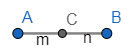Courses
Courses for Kids
Free study material
Free LIVE classes
MoreLIVE
Join Vedantu’s FREE Mastercalss

# If the line joining A(1, 3, 4) and B is divided by the point (-2, 3, 5) in the ratio 1:3, then B is:(a) (-11, 3, 8)(b) (-11, 3, -8)(c) (-8, 12, 20)(d) (13, 6, -13)Verified
358.8k+ views
Hint: In this question, we first need to draw the diagram with given conditions which gives a clear picture of what we have to find. Assume point B in terms of some variable and then solve for it using the two given points and the ratio by using the section formula.

$\left( \dfrac{{{m}_{1}}{{x}_{2}}+{{m}_{2}}{{x}_{1}}}{{{m}_{1}}+{{m}_{2}}},\dfrac{{{m}_{1}}{{y}_{2}}+{{m}_{2}}{{y}_{1}}}{{{m}_{1}}+{{m}_{2}}} \right)$

Let us look at some of the basic definitions
COORDINATE GEOMETRY:
The branch of Mathematics in which geometrical problems are solved through algebra by using the coordinate system, is known as coordinate geometry.
RECTANGULAR AXIS:
Let XOX' and YOY' be two fixed straight lines, which meet at right angles at O. Then,
XOX' is called the axis of X or the X-axis or abscissa.
Y'OY is called axis of Y or the Y-axis or ordinate.
The ordered pair of real numbers (x, y) is called Cartesian coordinate.
Coordinates of the origin are (0, 0).
The X-coordinate of a point on Y-axis is zero.
Y-coordinate of a point on the X-axis is zero.

QUADRANTS: The X and Y-axis divide the coordinate plane into four parts, each part is called a quadrant.
DISTANCE FORMULA: Distance between two points (x1 , y1) and (x2 , y2) , is
$\sqrt{{{\left( {{x}_{2}}-{{x}_{1}} \right)}^{2}}+{{\left( {{y}_{2}}-{{y}_{1}} \right)}^{2}}}$
Distance of the point (x1 , y1) from the origin is :
$\sqrt{{{x}_{1}}^{2}+{{y}_{1}}^{2}}$

SECTION FORMULA:
The coordinate of the point which divides the joint of (x1 , y1) and (x2 , y2) in the ratio m1:m2
internally, is:
$\left( \dfrac{{{m}_{1}}{{x}_{2}}+{{m}_{2}}{{x}_{1}}}{{{m}_{1}}+{{m}_{2}}},\dfrac{{{m}_{1}}{{y}_{2}}+{{m}_{2}}{{y}_{1}}}{{{m}_{1}}+{{m}_{2}}} \right)$
and externally is:
$\left( \dfrac{{{m}_{1}}{{x}_{2}}-{{m}_{2}}{{x}_{1}}}{{{m}_{1}}-{{m}_{2}}},\dfrac{{{m}_{1}}{{y}_{2}}-{{m}_{2}}{{y}_{1}}}{{{m}_{1}}-{{m}_{2}}} \right)$
Now, let us assume the coordinates of B as (x, y, z).
The ratio as m:n such that,
$m=1,n=3$$A=\left( 1,3,4 \right),C=\left( -2,3,5 \right)$
$\Rightarrow C=\left( \dfrac{m{{x}_{2}}+n{{x}_{1}}}{m+n},\dfrac{m{{y}_{2}}+n{{y}_{1}}}{m+n},\dfrac{m{{z}_{2}}+n{{z}_{1}}}{m+n} \right)$
$\Rightarrow C=\left( \dfrac{1\cdot x+3\cdot 1}{1+3},\dfrac{1\cdot y+3\cdot 3}{1+3},\dfrac{1\cdot z+3\cdot 4}{1+3} \right)$
$\Rightarrow C=\left( \dfrac{x+3}{4},\dfrac{y+9}{4},\dfrac{z+12}{4} \right)$
Now, on equating this with the coordinates of C we get,
$\Rightarrow \left( -2,3,5 \right)=\left( \dfrac{x+3}{4},\dfrac{y+9}{4},\dfrac{z+12}{4} \right)$
Now, on equating each coordinate respectively we get,
\begin{align} & \Rightarrow -2=\dfrac{x+3}{4} \\ & \Rightarrow -2\times 4=x+3 \\ & \Rightarrow x+3=-8 \\ & \Rightarrow x=-8-3 \\ & \therefore x=-11 \\ \end{align}
\begin{align} & \Rightarrow 3=\dfrac{y+9}{4} \\ & \Rightarrow 3\times 4=y+9 \\ & \Rightarrow y+9=12 \\ & \Rightarrow y=12-9 \\ & \therefore y=3 \\ \end{align}
\begin{align} & \Rightarrow 5=\dfrac{z+12}{4} \\ & \Rightarrow 5\times 4=z+12 \\ & \Rightarrow z+12=20 \\ & \Rightarrow z=20-12 \\ & \therefore z=8 \\ \end{align}
$\therefore B=\left( -11,3,8 \right)$
Hence, the correct option is (a).

Note: Instead of considering that the given point divides A and B internally if we consider that it divides them externally then the formula changes correspondingly and then substituting in it gives the different coordinates of B.
It is important to note that the C point divides A and B in the ratio 1:3 but not A and C are divided by B in the ratio 1:3. So, when we consider the section formula we substitute the value of coordinates A and B assuming some variable coordinates of B and then equate it to the given coordinates of C.
Last updated date: 23rd Sep 2023
Total views: 358.8k
Views today: 9.58k